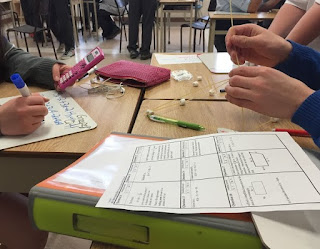## Monday, 25 January 2016

### Grade 11 Exam Review Tower ChallengeThis is a review activity on many of the topics found in grade 11 university where students answer questions and are rewarded with building materials for each correct answer. The building materials (spaghetti & marshmallows) are then used with the goal being the creation of tallest tower. This is based originally on a TIPS activity on quadratics for MBF3C (Unit 3, Day 6).

MCR3U
• demonstrate an understanding of functions, their representations, and their inverses, and make connections between the algebraic and graphical representations of functions using transformations;
• determine the zeros and the maximum or minimum of a quadratic function, and solve problems involving quadratic functions, including problems arising from real-world applications;
• demonstrate an understanding of equivalence as it relates to simplifying polynomial, radical, and rational expressions.
• evaluate powers with rational exponents, simplify expressions containing exponents, and describe properties of exponential functions represented in a variety of ways;
• identify and represent exponential functions, and solve problems involving exponential functions, including problems arising from real-world applications.
• demonstrate an understanding of the relationships involved in arithmetic and geometric sequences and series, and solve related problems;
• make connections between sequences, series, and financial applications, and solve problems involving compound interest and ordinary annuities.
• determine the values of the trigonometric ratios for angles less than 360º; prove simple trigonometric identities; and solve problems using the primary trigonometric ratios, the sine law, and the cosine law;
• demonstrate an understanding of periodic relationships and sinusoidal functions, and make connections between the numeric, graphical, and algebraic representations of sinusoidal functions;
• identify and represent sinusoidal functions, and solve problems involving sinusoidal functions, including problems arising from real-world applications.1. Place students in groups (ideally no bigger than 3 per group)
2. Hand out question sheets (and optional whiteboards) to each student.
3. Have students answer questions from their sheet in any order they want. For every correct answer they will get some building materials (eg: 2 spagetti & 3 marshmallows, the amount of each reward is indicated on the student question sheet ). The harder the question the more materials they will get. Eventually the building materials will be used to create a tower with the goal to create the tallest free standing tower.
4. Students work in groups to answer the questions and bring their solutions up to you to be checked. Only one member from each group can come up at a time. Each group can answer each question only once. To keep track of this, use the teacher answer sheet to check off which questions each group has answered as they come up.
5. Leave about 20 min at the end of the class for students to create their towers (students can no longer answer questions)
6. Take lots of pictures and celebrate the group with the tallest free standing tower.
• Gr11UTowerChallengeExamReview (with answer sheet) (pdfdoc)
Did you use this activity? Do you have a way to make it better? If so tell us in the comment section. Thanks